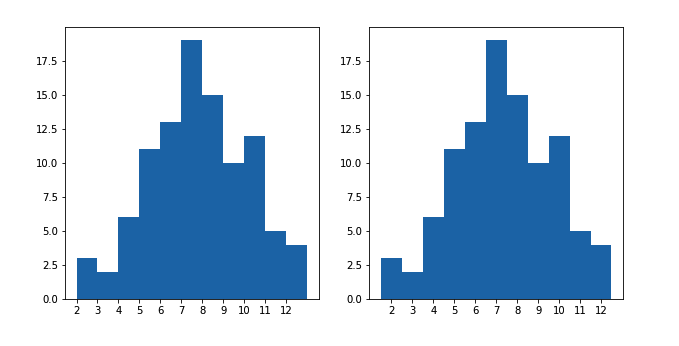# Python：matplotlib 之为离散数据选择图形 (二十九)

## 为离散数据选择图形

plt.figure(figsize = [10, 5])

# histogram on the left, bin edges on integers
plt.subplot(1, 2, 1)
bin_edges = np.arange(2, 12+1.1, 1) # note +1.1, see below
plt.hist(die_rolls, bins = bin_edges)
plt.xticks(np.arange(2, 12+1, 1))

# histogram on the right, bin edges between integers
plt.subplot(1, 2, 2)
bin_edges = np.arange(1.5, 12.5+1, 1)
plt.hist(die_rolls, bins = bin_edges)
plt.xticks(np.arange(2, 12+1, 1))bin_edges = np.arange(1.5, 12.5+1, 1)
plt.hist(die_rolls, bins = bin_edges, rwidth = 0.7)
plt.xticks(np.arange(2, 12+1, 1))